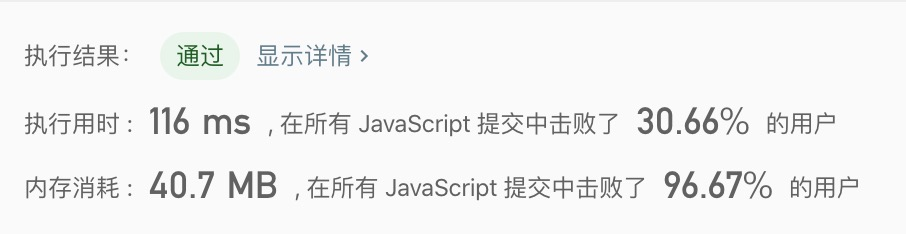Time Flying### 143.Reorder List

Given a singly linked list L: L0 → L1 → .. → Ln-1 → Ln, reorder it to: L0 → Ln → L1 → Ln-1 → L2 → Ln-2 →

You may `not modify the values in the list's nodes`, only nodes itself may be changed.

Example 1:

``Given 1->2->3->4, reorder it to 1->4->2->3.``

Example 2:

``Given 1->2->3->4->5, reorder it to 1->5->2->4->3.``

### Analyze

1. 步骤一: 找到链表中点后分割其为 left 链表、right 链表两部分;
2. 步骤二: 翻转 right 链表, 翻转链表思路同 206.Reverse_Linked_List;
3. 步骤三: 接着从 left 链表的左侧, 翻转后的 right 链表的左侧各取一个值进行交替拼接;

``````                   s               q
dummy -> 1 -> 2 -> 3 -> 4 -> 5 -> NULL

l
1 -> 2 -> 3 -> null

r
4 -> 5 -> NULL

l
1 -> 2 -> 3 -> null

r
5 -> 4 -> NULL

l
1 -> 5 -> 2 -> 3 -> NULL

r
4 -> NULL``````

`````` cur  next
1 -> 2 -> 3 -> null

prev cur
null <- 1 <- 2 -> 3 -> null

prev cur
null <- 1 <- 2 <- 3 -> null

prev
null <- 1 <- 2 <- 3 -> null``````
``````/**
* function ListNode(val) {
*     this.val = val;
*     this.next = null;
* }
*/
/**
*/
const dummy = new ListNode(0)

let slow = dummy
let quick = dummy

while (quick && quick.next) {
slow = slow.next
quick = quick.next
quick = quick.next
}

let right = slow.next
slow.next = null
let left = dummy.next

right = reverseList(right)

while (left && right) {
let lNext = left.next
let rNext = right.next
right.next = left.next
left.next = right
left = lNext
right = rNext
}

return dummy.next
}

var reverseList = (list) => {
let prev = null
let cur = list

while (cur) {
let next = cur.next
cur.next = prev

prev = cur
cur = next
}

return prev
}``````148(快慢指针)、234